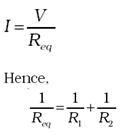### Combination of Resistors- Series

The current through a single resistor R across which there is a potential difference V is given by Ohm’s law I = V/R. Resistors are sometimes joined together and there are simple rules for calculation of equivalent resistance of such combination.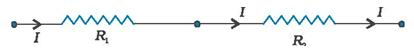Two resistors are said to be in series if only one of their end points is joined.

Consider two resistors R1 and R2 in series. The charge which leaves R1 must be entering R2. Since current measures the rate of flow of charge, this means that the same current I flows through R1 and R2. By Ohm’s law:

Potential difference across R1 = V1 = I R1, and Potential difference across R2 = V2 = I R2.

The potential difference V across the combination is V1+V2. Hence, V = V1+ V2 = I (R1 + R2).

This is as if the combination had an equivalent resistance Req, which by Ohm’s law is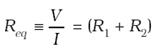### Combination of Resistors- Parallel

In parallel combination of two resistors, the charge that flows in at A from the left flows out partly through R1 and partly through R2. The currents I, I1, I2 shown in the figure are the rates of flow of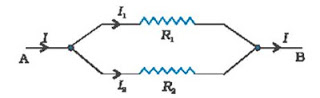Charge at the points indicated. Hence,The potential difference between A and B is given by the Ohm’s law applied to R1, V = I1 R1. Also, Ohm’s law applied to R2 gives V = I2 R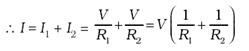If the combination was replaced by an equivalent resistance Req, by Ohm’s law